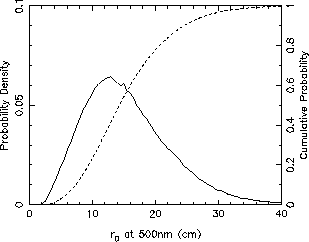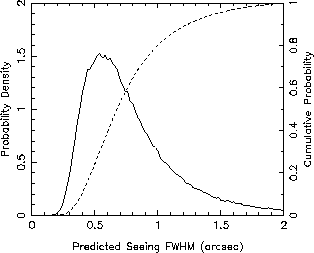# The Intrinsic Seeing Quality at the WHT Site

## The ING Differential Image Motion Seeing Monitor (DIMM)

 Contents Introduction Statistical results from the ING DIMM Astronomical seeing - the standard model How a DIMM works Introduction In order to estimate the intrinsic seeing, its contribution must be isolated from all other sources of image degradation  including telescope tracking errors, defocus and dome seeing. The differential image motion image monitor (DIMM) was developed specifically for this task.   The ING DIMM was supplied by Lhesa (Paris), and is mounted on a free-standing tower 75m from the WHT dome  (photograph of the ING DIMM). The principle of operation of the DIMM is described below. The DIMM measures the  strength of the aberrations due to atmospheric turbulence, and then predicts the seeing FWHM for a large telescope assuming the standard seeing model. The  aberration strength is parametrised by Fried's parameter (r0). Small values of r0 indicate strong turbulence, and hence poor seeing. Statistical results The ING DIMM was installed in October 1994 and an extensive database has been obtained,  so far comprising more than 250,000  seeing measurements on over 230 nights.  The statistical data are summarised in the following figures - distributions of all the DIMM  r0 and predicted image FWHM values measured between October 1994 and August 1998. Note that the FWHM predictions  are derived from the r0 values assuming that the telescope diameter is much greater than r0 but much smaller than the  outer scale of turbulence.  All values are corrected for zenith angle. The median predicted FWHM of 0.69 arcseconds shows that the intrinsic seeing at the WHT Site is excellent. A significant seasonal variation is found, with a median summer (May to September) value of 0.64 arcsec, and Winter median of 0.82 arcsec. DIMM surveys carried out by the Instituto de Astrofisica de Canarias at other locations on the La Palma site have yielded similar results (Muñoz-Tunón, et al, 1998).Distribution of Fried's parameter r0 measured with the ING DIMM seeing monitor, determined from 267379 values recorded on 233 nights between October 1994 and August 1998. The median value is r0 = 14.9cm.Distribution of predicted seeing FWHM values (0.98 lambda/r0) from the ING DIMM seeing monitor, for the same data set. The median value is FWHM = 0.69 arcsec. Astronomical seeing - the standard model   Starlight propagating through the Earth's atmosphere suffers random aberrations as it passes through regions where there is turbulent mixing of air of different temperatures and hence refractive indices. Atmospheric sounding experiments at La Palma and elsewhere have shown that such mixing typically occurs in a small number of layers, each a few tens of metres thick (Vernin, 1994). The strongest layers, in terms of the resulting optical distortion, are normally at low altitudes, with the majority of the seeing aberrations typically originating from less than 2km above the telescope. At the focus of a large telescope the effect of these aberrations is to form a rapidly changing 'speckle' image. The long exposure PSF  is then the co-addition of a large number of random speckle realisations, resulting in an approximately Gaussian PSF with FWHM typically in  the range 0.5 to 2 arcseconds at a good observing site. The standard model for astronomical seeing, developed largely by Tatarski (1961) and Fried (1965), is based on the work of Kolmogorov (1941) on atmospheric turbulence. The analysis has been reviewed in detail by Roddier (1981).  The crucial result is that for propagation through turbulence in the Kolmogorov model the structure function  Dp(r) of the wavefront phase perturbations p(r)  at ground level scales as separation r to the 5/3 power: Dp(r)  =  <[p(r') - p(r'-r)]2>  =  6.88 (|r|/r0)5/3  where the scaling length r0 is known as Fried's parameter, and is a measure of the strength of the seeing distortions. For this structure function the seeing limited FWHM of the long exposure PSF for a telescope with diameter much larger than r0 is given by: FWHM =  0.98 lambda / r0 where lambda is the wavelength of observation. Since r0 scales as (lambda)6/5 , the image FWHM has only a weak (lambda-1/5) dependence on wavelength. The typical size of the Fried length at a good observing site is 10cm at 500nm, which yields a long exposure image width of approximately 1 arcsecond. It is important to note that the  observed image FWHM will be equal to that predicted by this equation only if there is no contribution to image width from other sources such as telescope focus or tracking errors. For the theoretical Kolmogorov/Tatarski structure function,  seeing distortions of the wavefront extend to infinitely large spatial scales.  In reality an upper limit is imposed by the finite thickness of the contributing turbulent layers. Hence the 5/3 scaling will apply only to spatial scales smaller than an upper limit known as the  outer scale of turbulence L0.  If the outer scale is not much larger than the telescope aperture diameter, then the image FWHM will be smaller than 0.98 lambda / r0, particularly at long wavelengths. How a DIMM works The principle of the DIMM is to measure the variance of the differential centroid motion for images of a star produced separately from two apertures of known separation within the entrance pupil of a telescope. The differential image motion is unaffected by tracking errors or telescope shake, or by small focus errors, and so gives an unbiased estimate of the image degradation due to the free atmosphere alone. The ING DIMM  is based upon a 20cm Celestron telescope with its entrance pupil  masked to form two apertures with diameter 60mm and separation 140mm.  A wedge prism covers one aperture so that two separated images of a bright star are produced at the telescope focus, where they are recorded by an intensified CCD camera.  A frame rate of 25Hz allows the image motions to be recorded.  The instrument is mounted on an open tower approximately 100 metres from the WHT building, so that dome seeing and low level ground-to-air seeing effects are also avoided. For the Tatarski spectrum of wavefront phase fluctuations, the variance of the differential image motion in the direction parallel to the aperture alignment sl2 is related to r0  by sl2 = 2  lambda  r0-5/3  [0.179 d-1/3  -  0.0968  r-1/3 ]  where d is the diameter of the apertures and r their separation. A similar equation gives the orthogonal component of the differential image motion st2. The variances sl2 and st2  are estimated from the measured image motions (typically averaged for 15 seconds), and a value for r0 is obtained from the equation above. A prediction of the long exposure image FWHM is then given by  0.98 lambda / r0. The uncertainty of the seeing estimates, determined mainly by photon noise, is typically less than 5 per cent. The seeing values are corrected for zenith distance z, using the formula: r0(zenith) = r0(z)  sec(z)0.6 The DIMM FWHM prediction assumes that the spatial spectrum of wavefront aberrations is well described by the Tatarski structure function.  Furthermore, measurements are made only on the 140mm baseline which separates the DIMM apertures - a much smaller scale than the 4.2m aperture of the WHT. The accuracy of the DIMM seeing estimates for the WHT is therefore dependent on the validity of the Kolmogorov turbulence model for the spatial scales of interest. In particular, the DIMM will over-estimate the seeing for the WHT  if the outer scale of turbulence is not much larger than the WHT aperture size. References: Fried, D.L., 1965, J. Opt. Soc. Am., 55, 1427. Kolmogorov, A.N., 1941, in Tikhomirov, V. M., ed, Selected works of A.N. Komogorov, Mathematics and its applications (Soviet series), Klewer Academic press (1991). Muñoz-Tunón,  C., Varela, A.M., Mahoney, T., 1998, New Astronomy Reviews, 42, 409. Roddier, F., 1981, in Wolf E., ed, Progress in Optics 19, North Holland, Amsterdam. Tatarski, V.I., 1961, Wavefront Propagation in a Turbulent Medium, Dover, New York. Vernin, J.,  &  Muñoz-Tunón,  C., 1994,  AA, 284,  311.